# Analytical Studies on the Hydraulic Performance of Chevron Type Plate Heat Exchanger

Analytical Studies on the Hydraulic Performance of Chevron Type Plate Heat Exchanger

Bhupal Kumar S.N. Singh

Research scholar, HT Lab, Deptt. of mechanical engineering, ISM Dhanbad, Jharkhand, India, 826004

Associate professor, Deptt., of mechanical engineering, ISM Dhanbad. Jharkhand, India 826004

Page:
17-24
|
DOI:
https://doi.org/10.18280/ijht.330103
N/A
|
Accepted:
N/A
|
Published:
31 March 2015
| Citation

OPEN ACCESS

Abstract:

The results of analytical studies on the hydraulic performance of chevron type plate heat exchanger  for  different  aspect ratios, Reynolds number, maldistribution parameters, no of channels, fixed port size, and flow rates using water as the working fluid in the channels have been presented. Some analytical  results  have  been validated with the experimental  results available in the literature. Pressure drop in the heat exchanger is found to depend on flow rate, numbers of channels and aspect ratio. Friction factor in a channel also depends on flow rate of fluid and aspect ratio. The flow distribution and pressure drop in the U-type arrangement plate heat exchangers are studied for a wide range of Reynolds number (200-5800). The flow maldistribution brings about an increase in pressure drop across the heat exchanger.

Keywords:

Plate heat exchanger, maldistribution, chevron, flow distribution, pressure drop, process.

1. Introduction

Plate and frame heat exchangers are offered by a large number of manufacturers as standard series production equipment over a wide range of sizes. They consist of a number of gasketed metal plates clamped between a stationary head and a follower plate by tie bolts. In recent times, the plate heat exchangers are extensively used for heating, cooling, heat-regeneration and chemical processing industries due to its favorable characteristics, such as high overall heat transfer coefficients, easy maintenance, compact size, convenience to increase the heat transfer area, suitability in hygienic application and easy of  cleaning.

Mehrabian and Poulter  studied the local hydrodynamic and thermal characteristics of the flow between two identical APV SR3 plates and looks at the effect of corrugation angle on the performance when the plate spacing is fixed. Galeazzo et al. studied the virtual prototype of a four-channel plate heat exchanger with flat plates was developed using computational fluid dynamics (CFD). Parallel and series flow arrangements were tested and experimental results were compared to numerical predictions for heat load obtained from the 3D CFD model and also from a 1D plug-flow model. Tsai et al.  investigated the hydrodynamic characteristics and distribution of flow in two cross-corrugated channels of plate heat exchangers. The velocity, pressure and flow distribution of the fluid among the two channels of the plate heat exchanger have also been presented. Pahlavanzadeh et al.  investigated the effect of two tube inserts (wire coil and wire mesh) on the heat transfer enhancement, pressure drop and mineral salts fouling mitigation in tube of a heat exchanger. Bobbili et al.  have been found out the flow and the pressure difference across the port to channel in plate heat exchangers for a wide range of Reynolds number, 1000–17000. The studied low corrugation angle plates have been used for different number of channels, namely, 20 and 80. Fernandes et al.  have been numerically studied the geometry of the channels for laminar flows of Newtonian and power-law fluids through cross-corrugated chevron type plate heat exchangers (PHEs). The plates area enlargement factor was a typical one (1.17), the corrugation angle, β, varied between $30^{\circ}$ and $60^{\circ}$ and the flow index behavior, n, between 0.25 and 1. Han et al.  have been obtained the three dimensional temperature, pressure, and velocity fields by using the chevron corrugated-plate heat exchanger and results is simulated. Rao and das  studied the influence of flow maldistribution on the pressure drop across a plate heat exchanger. Bassiouncy and Martin  studied the axial velocity and pressure distributions in both the intake and exhaust conduits of plate heat exchangers, the flow distribution in the channels between the plates and the total pressure drop. Naik and Matawala  studied the effect of variation of chevron angles with other geometric parameter on the heat transfer coefficient and for using the wide range of Reynolds number, 50–10000. Fernandes et al.  have been studied the corrugation angle and channel aspect ratio of the passages vary in a broad range, PHEs with common area enlargement factors and with high area density. The tortuosity coefficient and the coefficient K (Kozeny’s coefficient in granular beds) from the friction factor correlations increase with the increase of the channels aspect ratio and the decrease of the chevron angle.

After critical review of the literatures, it is clear that works on maldistribution in a chevron type plate heat exchanger have not been done in detail. The present analytical study aims to bring out a clear picture about how the pressure drop is affected by the flow maldistribution in U-type PHE in presence of a large number of channels per fluid, varying channel aspect ratio and wide range of Reynolds number.

2. Plate Geometry

The thermal-hydraulic performance of plate heat exchanger is strongly dependent on the geometrical properties of the chevron plates [11-12], namely on the corrugation angle, β, area enlargement factor, φ, defined as the ratio between the effective plate area and projected plate area, and channel aspect ratio (Fig. 1).

## 1.png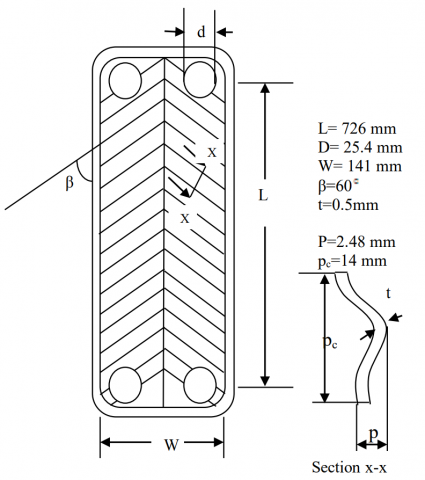Figure 1. Geometric characteristics of plate

3. Mathematical Analysis

All the data have been found out under steady state conditions and the range of operating flow rate were taken to have Reynolds number from 200 to 5800 for U type plate heat exchanger. The friction factor and pressure drop have been obtained for a single channel. The correlation has been used for obtaining the channel friction factor as

$f_{c h}=4291 R e^{-1.278}+0.3343$       (1)

Mean Velocity of fluid in channel as

$u_{c h}=\frac{v}{w b n}$  (2)

It must be mentioned here that the Reynolds number for plate heat exchanger is defined on the basis of twice the plate spacing b, as

$R e=\frac{u_{c h}}{v}\left(D_{h}\right)$  (3)

The pressure drop in inlet/outlet ports of the channels, the pressure drop in the short length (1 m) of the connecting pipe (ID 30.48 mm), and the pressure drop in the main corrugated flow passage of the plate package. Hence, the total pressure drop (including the port to channel pressure drop) can be obtained from

$\Delta P_{t p}=\Delta P_{c m}+\Delta P_{p o r t}+\Delta P_{e c}+\Delta P_{f, p i p e}$    (4)

The port pressure drop is calculated based on the total flow rate by using an empirical established equation 

$\Delta P_{\text {port }}=1.5 \rho \frac{v^{2} v}{2}$    (5)

The pressure drops due to bend, sudden contraction and sudden expansion at inlet and outlets, respectively, have been calculated by using the following formula:

$\Delta P_{e c}=K_{e c} \rho \frac{v^{2} v}{2}$      (6)

Where $K_{e c}$ is the total pressure loss coefficient of the bend, sudden contraction and sudden expansion at the inlet and outlet of the ports. The pressure drop due to friction in the small steel connecting pipe at the inlet and outlet of the plate heat exchanger was estimated on the basis of the smooth steel tube friction factor and pipe flow velocity as

$\Delta P_{f, p i p e}=f_{p i p e} \frac{L_{p i p e}}{d_{p i p e}} \rho \frac{V^{2} p i p e}{2}$   (7)

The pressure drop due to friction in the corrugated passage is calculated based on the flow rate by using an empirical formula:

$\Delta P_{c h m}=f_{c h} \frac{L_{c h}}{d_{h}} \rho \frac{v^{2} c h}{2}$     (8)

The value of m2 is calculated by Bassiouny and Martin  equation for identical inlet and outlet port dimension

$m^{2}=\left(\frac{n A_{C}}{A_{P}}\right)^{2} \frac{1}{\xi_{c}}$     (9)

Here $\xi_{c}$ is the overall frictional resistance of the channel and is equal to $\xi_{c}=f L_{c h} / d_{h}$ + other minor losses including turning loss. The total dimensionless pressure of the plate heat exchanger is

$P_{0}-P_{0}^{*}=\left(\frac{m^{2}}{\tanh ^{2} m}\right)\left(\frac{A_{P}}{n A_{c}}\right)^{2} \frac{\xi_{c}}{2}$   (10)

The value of the flow maldistribution parameter, m2 has been used to obtain the pressure drop distribution from the first to last channel by using the following equation given by Bassiouny and Martin :

$\frac{\Delta P}{\rho w_{o}^{2}}=\left(\frac{A_{p}}{n A_{c}}\right)^{2} \frac{\xi_{c}}{2} m^{2} \frac{(\cosh m(1-z))^{2}}{\left(\sinh m_{1}\right)^{2}}$     (11)

Applying the boundary conditions as one obtain in the final equation for port to channel flow distribution as given by Bassiouny and Martin 

$u_{c}=\left(\frac{A_{P}}{n A_{c}}\right) m \frac{\cosh m(1-z)}{\sinh m}$       (12)

Table 1. Range of parameter considered in the present study

 Parameters Range Reynolds number, Re 200-5800 Aspect ratio, A 145.2-363 Maldistribution parameter, m2 1.00009E-09-16.289 Flow rate (L/sec) 0.139-6.25 Number of channels per fluid 7-19 Port size (mm) 25.4-30.48

4. Results and Discussion

Table 1 shows the range of parameters considered in the present study. On the basis of the finding, the present work is aimed at analytical study the flow maldistribution parameters, m2 with pressure drop in plate heat exchangers for use in wide range of Reynolds number and aspect ratio.

4.1 Validation

Validation with experimental results of Rao and Das 

Fig. 2 shows the comparison of present analytical results of non dimensional pressure drop with the experimental results of Rao and Das  for the channels of U type plate heat exchanger.  Both the experimental and present results are found in good agreement and both the results are within 10%. It is seen from figure that the pressure drops is maximum in 10 channels due to increase the channel velocity. From this Figure 2, it is observed that the non dimensional pressure drop decreases with increasing channel Reynolds number because the non dimensional velocity, uc, itself increases for a fixed number of channels.

## 2.png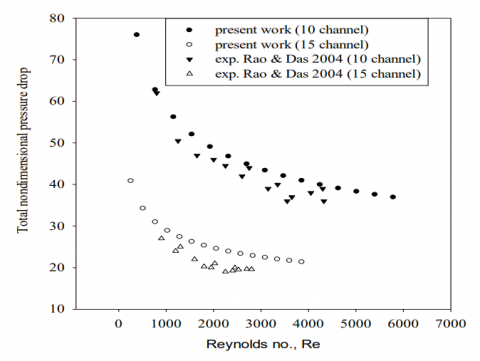Figure 2. Variation of total non dimensional pressure drop with Reynolds number

## 3.png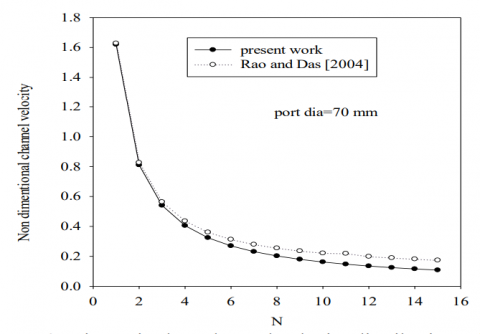Figure 3. Dimensionless channel velocity distribution with no. Of channel for U type

Validation with analytical results of Rao and Das 

Fig. 3 shows the variation of non dimensional channel velocity with number of channel. It shows that a channel velocity decrease with increase in number of channel. It is also seen from figure that the channel velocity is higher at minimum number of channel because of constant flow rates in the heat exchanger. There is a good agreement between the present and the analytical results .

4.2 Typical results for chevron type PHE

Variation of channel friction with Reynolds number

Fig. 4 shows the variation of channel friction factor with Reynolds number for three different channel arrangements (7, 13, and 19) and fixed channel aspect ratio. Fig. 4 reveals that channel friction factor decreases with increase in Reynolds number and the loss friction factor in case of seven number of channel is smaller than that of thirteen and nineteen no of channel for same rate of water flow in plate heat exchanger because the channel flow velocity is increased in channel.

Variation of total pressure drop with Reynolds number

Fig. 5 shows the variation of total pressure drop with Reynolds number for different number of channels arrangement (7, 13 and 19) and fixed aspect ratio (A=363). From Fig. 5, it is observed that as the number of channel increases, pressure drop increases due to maldistribution. The increase in pressure drop is more for large number of channels because maldistribution is a strong function of number of channels.

## 4.png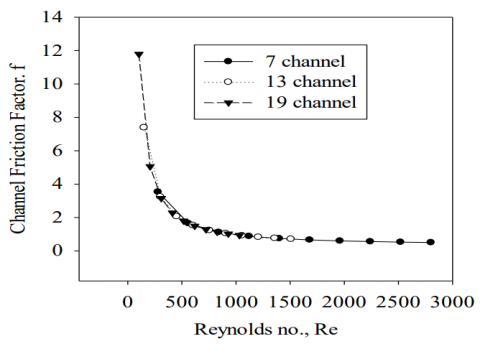Figure 4. Variation of channel friction factor with Reynolds no

Variation of the flow maldistribution parameter, m2 with Reynolds number

Fig. 6 shows the variation of the flow maldistribution parameter, m2 with Reynolds number for 7, 13 and 19 channel U-type plate heat exchanger. At the small number of channel (7 channel), the m2 value does not change with the flow rates appreciably and the value are close to zero indicating a uniform flow through the channel. From Fig. 6, it is observed that the flow maldistribution m2increases with increase the number of channel for same flow rate of water in U type plate heat exchanger because the flow maldistribution parameters, m2 is a function of number of channel and channel velocity. The larger value of m2 shows the non uniform flow in the channel.

Variation of channel velocities with the number of channel

Fig. 7 shows the variation of distribution of the channel velocities with the number of channel for two different diameter of port size in U-type plate heat exchanger. As seen from Fig.7, channel velocity increases with increasing port diameter for fixed aspect ratio (A=363) because of port diameter is directly influenced the channel velocity in plate type heat exchanger. The channel velocity is decreased with increase of number of channels due to increase in pressure drop for large number of channels. It can be also seen from Figure 7 that the channel flow velocity is almost constant for increasing the number of channels.

Variation of total pressure drop with channel mass flow rate

Fig. 8 shows the variation of total pressure drops with channel mass flow rate for three different numbers of channels. As seen from Figure 8 pressure drop is increases with increasing the mass flow rate for different number of channels because of high turbulence at high mass flow rate.  It is observed that the more pressure drop for smaller number of channel at the same mass flow rate in plate heat exchanger because maldistribution is a strong function of number of channels.

Variation of m2 with Reynolds number

Fig. 9 shows the variation of flow maldistribution with Reynolds number for three different aspect ratios. At increase the aspect ratio, the values of flow maldistribution parameters, m2 does not change with Reynolds number appreciably and the values are near to zero indicating a uniform flow distribution. As seen from Figure 9 that the increase in flow maldistribution parameter, m2 is increased with decreases the aspect ratio because maldistribution is a strong function of hydraulic diameter.

Variation of pressure drop in channel with Reynolds number

Fig. 10 shows the variation of pressure drop in channel with Reynolds number for three different aspect ratios. The pressure drop is increased with increase of Reynolds number for all aspect ratio values. The channel pressure drop is a maximum at the highest aspect ratio value because the disturbance creates in flow path in channels.

Comparison of channel non dimensional pressure drop with number of channel

Fig. 11 shows a comparison of channel non dimensional pressure drop with number of channels for different aspect ratios. As seen from Fig. 11 that the non dimensional pressure drop is decreased with increase the number of channel for three different aspect ratios due to channel velocity is increased. The pressure drop increased with increase the aspect ratio because of hydraulic diameter is decreased.

## 5.pngFigure 5. Variation of total pressure drop with Reynolds no

## 6.pngFigure 6. Variation of flow maldistribution with Reynolds no

Variation of flow maldistribution with Reynolds number

The Fig. 12 shows the variation of flow maldistribution in the channel with Reynolds number for three different aspect ratio (363, 242 and 145.2) and three different numbers of channels (7, 13, and 19). Figure 12 shows that the flow maldistribution parameter, m2 is decreased with increase the aspect ratios because the pressure drop in channel is increased. These changes are very severe in a 145.2 aspect ratio and it is also shown that the flow maldistribution parameter, m2 varies from the number of channels viz. 7, 13, and 19 channels. These changes are very severe in a 19 channels whereas for small no of plate channels and larger value of aspect ratio, the flow maldistribution is very small.

## 7.png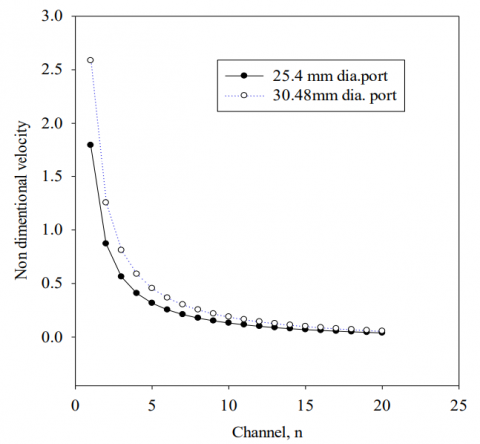Figure 7. Variation of non dimensional velocity with channels

## 8.png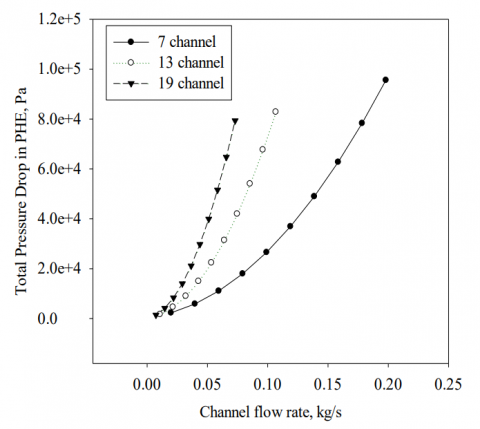Figure 8. Variation of total pressure drop with channel flow rate

Variation of pressure drop in channel with Reynolds number

Fig. 13 shows the variation of pressure drop in channel with Reynolds number for different channel aspect ratios and number of channels. As seen from Figure 13 that the pressure drop is increased with increase the Reynolds number for different aspect ratios and number of channel arrangement due to maldistribution is a function of number of channels and aspect ratios. It can also seen from Fig that the variation of pressure drop with Reynolds number is almost same for different aspect ratios and number of channels.

## 9.png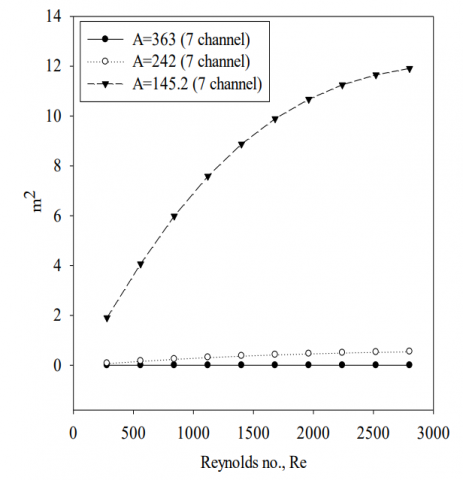Figure 9. Variation of m2 with Reynolds no

## 10.pngFigure 10. Variation of pressure drop in channel with Reynolds no

## 11.png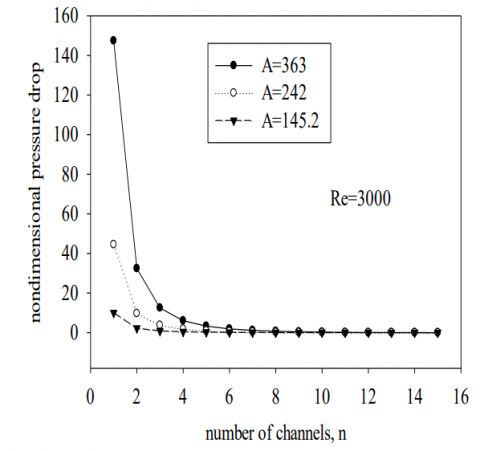Figure 11. Comparison of channel non dimensional pressure drop with number of channel

## 12.png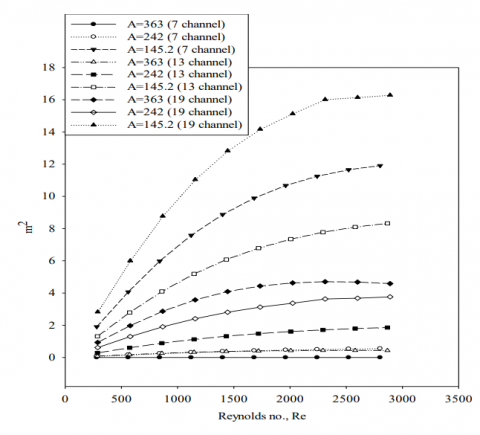Figure 12. Variation of flow maldistribution with Reynolds no

## 13.png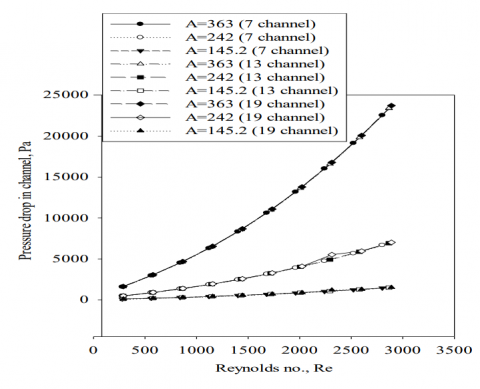Figure 13. Variation of pressure drop in channel with Reynolds no

5. Conclusions

The analytical results show for a plate heat exchanger is presented for wide range of Reynolds number. A detailed study has been presented to investigate the pressure drop across a plate heat exchanger for channel flow maldistribution. The pressure drops and flow maldistribution parameter, m2 in a chevron type plate heat exchanger with different aspect ratio, different number of channel, and water flow rate were studied. The Reynolds number increases with decrease the friction factor in the channel. The total pressure drop across the heat exchanger is found to critically depend on flow rate and aspect ratio. The non dimensional velocity across the channel is found to depend on flow rate as well as port size. There is no much influence of flow maldistribution parameter, m2 on channel flow for small number of channel. The pressure drop in channel is increased with increase the aspect ratio and number of plate packages. It is found that for a given aspect ratio the pressure drop decreases with increase the water flow rate, consequently increase the flow maldistribution parameter in the channel. The predictions are in accordance with experimental and analytical results for U type configuration. The Results from this work will be useful for the design of heat exchangers for wide range of mass flow rate of fluid.

Nomenclature
 A aspect ratio, (L/b) Ap cross- sectional area of the port, (m2) Ac cross- sectional area of the channel, (m2) b plate spacing, (m) Dh hydraulic diameter of the channel, (m) dpipe connection pipe diameter, (m) f Fanning friction factor fch channel friction factor kec total pressure loss coefficient due to sudden expansion, sudden contraction and bend L vertical distance between the two ports, (m) m2 flow maldistribution parameter n number of channels per fluid pc corrugation pitch, (m) $\Delta$pchm mean channel pressure drop, (pa) $\Delta$pec pressure drop due to sudden expansion and  contraction, (pa) $\Delta$pfirst pressure drop at the first channel, (pa) $\Delta$pport pressure drop between the inlet and outlet ports in PHE, (pa) $\Delta$p dimensionless pressure drop across a plate heat Exchanger Re Reynolds number, Re=$\left(\rho u_{c} D_{h}\right) / \mu$ t thickness of plate, m $u_{c}$ dimensionless channel velocity W width of the plate, m Greek letters $\beta$ corrugation angle of the plate ($\circ$ ) Φ area enlargement factor $\rho$ density of the fluid, (kg/m3) ξc overall friction coefficient of the corrugated channel
References

1. M.A. Mehrabian and R. Poulter, Hydrodynamics and thermal characteristics of corrugated channels: computational approach, Applied Mathematical Modeling, vol. 24, pp. 343-364, 2000.

2. Flavio C.C. Galeazzo, Raquel Y. Miurab, Jorge A.W. Gut, Carmen C. Tadini, Experimental and numerical  heat transfer in a plate heat exchanger, Chemical Engineering Science, vol. 61, pp. 7133 – 7138, 2006.

3. Ying-Chi Tsai, Fung-BaoLiu, Po-TsunShen, Investigations of the pressure drop and flow distribution in a chevron-type plate heat exchanger, International Communications in Heat and Mass Transfer, vol. 36, pp. 574–578, 2009.

4. H. Pahlavanzadeh, M.R. Jafari Nasr, S.H. Mozaffari, Experimental study of thermo-hydraulic and fouling performance of enhanced heat exchangers, International Communications in Heat and Mass Transfer, vol. 34, pp. 907–916, 2007.

5. Prabhakara Rao, Bobbili, BengtSunden, Sarit K.  Das, An experimental investigation of the port flow maldistribution in small and large plate package heat exchangers, Applied Thermal Engineering, vol.26, pp. 1919–1926, 2006.

6. Carla S. Fernandes, Ricardo P. Dias, João M. Nóbrega, João M. Maia, Friction factors of power-law fluids in chevron-type plate heat exchangers, Journal of Food Engineering, vol.89, pp. 441–447, 2008.

7. Xiao-Hong Han, Li-Qi Cui, Shao-Jie Chen, Guang- Ming Chen, Qin Wang, A numerical and experimental study of chevron, corrugated-plate heat exchangers, International Communications in Heat and Mass Transfer, vol. 37, pp. 1008–1014, 2010.

8. B. PrabhakaraRao and Sarit K. Das, An experimental study on the influence of flow maldistribution on the pressure drop across a plate heat exchanger. ASME 126, 680, 2004.

9. M. K.Bassiouny and H. Martin, Flow distribution and pressure drop in plate heat exchangers-I, Chemical Engineering Science, vol. 39, pp. 693-700, 1984.

10. Vishal R. Naik and V.K. Matawala, experimental investigation of single phase chevron type plate heat exchanger, International Journal of Engineering and Advanced Technology, vol. 2, pp. 2249-8958, 2013.

11. Carla S. Fernandes, Ricardo P. Dias, João M. Nóbrega, João M. Maia, Laminar flow in chevron-type plate heat exchangers: CFD analysis of tortuosity, shape factor and friction factor, Chemical Engineering and Processing, vol. 46, pp.825–833, 2007.

12. R.K. Shah, W.W. Focke, plate heat exchangers  and  their design theory, in: R.K Shah et al.(Eds.), Heat Transfer Equipment Design, Hemisphere, Washington, pp. 227-254, 1988.

13. D.Missirlis, M.Flouros and K.Yakinthos, Heat transfer and flow field investigation of heat exchanger for aero engine application, International Journal of Heat and Technology, vol.29, pp. 57-64, 2011.

14. P.K. Sarma, D. Radhakrishna, V. Srinivas, V. Dharma rao, C.P. Ramnaryanan, An analytical approach  to  solve a cross flow heat exchanger for unmixed hot and cold media, International Journal of Heat and Technology, vol. 29, pp. 113-118, 2011.